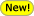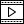## Algorithms

SPMF offers implementations of the following data mining algorithms.

### Sequential Pattern Mining

These algorithms discover sequential patterns in a set of sequences. For a good overview of sequential pattern mining algorithms, please read this survey paper.

### Sequential Rule Mining

These algorithms discover sequential rules in a set of sequences.

### Sequence Prediction

These algorithms for predicting the next symbol of a sequence based on a set of training sequences

### Itemset Mining

These algorithms discover interesting itemsets (sets of values) that appear in a transaction database (database records containing symbolic data). For a good overview of itemset mining, please read this survey paper.

### Episode Mining

These algorithms discover patterns (episodes) that appear in a single sequence of events.

• algorithms for mining frequent episodes
• the EMMA algorithm, which finds the frequent episodes, and counts the support based on the head frequency (Kuo-Yu et al., 2008
• the AFEM algorithm, which finds the frequent episodes, and counts the support based on the head frequency (Fournier-Viger et al., 2022• the MINEPI+ algorithm, which finds the frequent episodes, and counts the support based on the head frequency (Kuo-Yu et al., 2008
• the MINEPI algorithm, which finds the frequent episodes, counts the support based on minimal occurrences, and does not allow simultaneous events (Mannila & Toivonen, 1997
• algorithms for mining the top-k frequent episodes
• the TKE algorithm, which finds the top-k most frequent episodes based on the head frequency (Fournier-Viger et al., 2020)• algorithms for mining maximal frequent episodes
• the MaxFEM algorithm, which finds the maximal frequent episodes, and counts the support based on the head frequency (Fournier-Viger et al., 2022 , powerpoint)• algorithms for mining episode rules
• algorithms for mining high utility episodes in a sequence of complex events (a transaction database) with utility information
• the HUE-SPAN algorithm (Fournier-Viger et al., 2019, powerpoint) for mining high utility episodes in a sequence of complex events (a transaction database) with utility information• the US-SPAN algorithm (Wu et al., 2013 ) for mining high utility episodes in a sequence of complex events (a transaction database) with utility information
• the TUP algorithm (Rathore et al., 2016) for mining the top-k high utility episodes in a sequence of complex events (a transaction database) with utility information
• algorithms for mining nonoverlapping sequential patterns in one or many sequences of symbols
• the NOSEP algorithm (Wu et al., 2018)### Periodic Pattern Mining

These algorithms discover patterns that periodically appear in a sequence of records (e.g. transactions)

• Algorithms for finding periodic patterns in a single sequence of complex events (also called a transaction database)
• the PFPM algorithm (Fournier-Viger et al, 2016a, powerpoint, video) for mining frequent periodic patterns in a sequence of transactions (a transaction database))
• the SPP-Growth algorithm (Fournier-Viger et al. 2019, powerpoint, video) for mining stable periodic patterns in a transaction database with or without timestamps
• the TSPIN algorithm to discover the Top-k Stable Periodic frequent itemset (Fournier-Viger et al.,2021 )• the LPP-Growth algorithm (Fournier-Viger. 2020, powerpoint) for mining locally periodic patterns in a transaction database with or without timestamps.• the LPPM_breadth algorithm (Fournier-Viger. 2020, powerpoint) for mining locally periodic patterns in a transaction database with or without timestamps.• the LPPM_depth algorithm (Fournier-Viger. 2020, powerpoint) for mining locally periodic patterns in a transaction database with or without timestamps.• the PHM algorithm (Fournier-Viger et al, 2016b, powerpoint) for mining periodic high-utility patterns (periodic patterns that yield a high profit) in a sequence of transactions (a transaction database) containing utility information
• the PPFP algorithm to discover productive periodic frequent itemsets in a transaction database (Nofong, V. M., 2016)• the NPFPM algorithm to discover non-redundant periodic frequent itemsets in a transaction database (Afriyie et al., 2020, 2021)• the SRPFPM algorithm to discover self-reliant periodic frequent patterns in a transaction database (Nofong et al, 2021)• Algorithms for finding periodic patterns in multiple sequences of events

### Graph Pattern MiningThese algorithms discover patterns in graphs

• Algorithms for mining patterns in a database of labelled graphs
• the TKG algorithm for mining the top-k frequent subgraphs in a graph database (Fournier-Viger, 2019, powerpoint)• the gSpan algorithm for mining all the frequent subgraphs in a graph database (Yan et al., 2002)
• the cgSpan algorithm for mining all the frequent closed subgraphs in a graph database (using the traditional support or MNI support) (Shaul et al. 2021)• Algorithms for mining patterns in a dynamic attributed graph

### High-Utility Pattern Mining

These algorithms discover patterns having a high utility (importance) in different kinds of data. For a good overview of high utility itemset mining, you may read this survey paper, and the high utility-pattern mining book.

### Association Rule Mining

These algorithms discover interesting associations between symbols (values) in a transaction database (database records with binary attributes).

• an algorithm for mining all association rules in a transaction database (Agrawal & Srikant, 1994)• an algorithm for mining all association rules with the lift measure in a transaction database (adapted from Agrawal & Srikant, 1994)
• an algorithm for mining the IGB informative and generic basis of association rules in a transaction database (Gasmi et al., 2005)
• an algorithm for mining perfectly sporadic association rules (Koh & Roundtree, 2005)
• an algorithm for mining closed association rules (Szathmary et al. 2006).
• an algorithm for mining minimal non redundant association rules (Kryszkiewicz, 1998)
• the Indirect algorithm for mining indirect association rules (Tan et al. 2000; Tan et 2006)
• the FHSAR algorithm for hiding sensitive association rules (Weng et al. 2008)
• the TopKRules algorithm for mining the top-k association rules (Fournier-Viger, 2012b, powerpoint)
• the TopKClassRules algorithm for mining the top-k class association rules (a variation of TopKRules. This latter is described in Fournier-Viger, 2012b, powerpoint)
• the TNR algorithm for mining top-k non-redundant association rules (Fournier-Viger 2012d, powerpoint)
• the HGB and HGB_All algorithm to derive all high utility association rules or just the non redundant high utility association rules (Sahoo et al., 2015)### Stream pattern mining

These algorithms discovers various kinds of patterns in a stream (an infinite sequence of database records (transactions))

• the estDec algorithm for mining recent frequent itemsets in a data stream (Chang & Lee, 2003)
• the estDec+ algorithm for mining recent frequent itemsets in a data stream (Shin et al., 2014)
• the CloStream algorithm for mining frequent closed itemsets in a data stream (Yen et al, 2009)
• algorithms for mining the top-k high utility itemsets from a data stream with a window

### Clustering

These algorithms automatically find clusters in different kinds of data

• the original K-Means algorithm (MacQueen, 1967)
• the Bisecting K-Means algorithm (Steinbach et al, 2000)
• algorithms for density-based clustering
• the DBScan algorithm (Ester et al., 1996)
• the Optics algorithm to extract a cluster ordering of points, which can then be use to generate DBScan style clusters and more (Ankerst et al, 1999)
• a hierarchical clustering algorithm
• a tool called Cluster Viewer for visualizing clusters
• a tool called Instance Viewer for visualizing the input of clustering algorithms

### Time series mining

These algorithms perform various tasks to analyze time series data

• an algorithm for converting a time series to a sequence of symbols using the SAX representation of time series. Note that if one converts a set of time series with SAX, he will obtain a sequence database, which allows to then apply traditional algorihtms for sequential rule mining and sequential pattern mining on time series (SAX, 2007).
• algorithms for calculating the prior moving average of a time series (to remove noise)
• algorithms for calculating the cumulative moving average f a time series (to remove noise)
• algorithms for calculating the central moving average of a time series (to remove noise)
• an algorithm for calculating the median smoothing of a time series (to remove noise)
• an algorithm for calculating the exponential smoothing of a time series (to remove noise)
• an algorithm for calculating the min max normalization of a time series
• an algorithm for calculating the autocorrelation function of a time series
• an algorithm for calculating the standardization of a time series
• an algorithm for calculating the first and second order differencing of a time series
• an algorithm for calculating the piecewise aggregate approximation of a time series (to reduce the number of data points of a time series)
• an algorithm for calculating the linear regression of a time series (using the least squares method)
• an algorithm for splitting a time series into segments of a given length
• an algorithm for splitting a time series into a given number of segments
• algorithms to cluster time series (group time-series according to their similarities). This can be done by applying the clustering algorithms offered in SPMF (K-Means, Bisecting K-Means, DBScan, OPTICS, Hierarchical clustering) on time series.
• a tool called Time Series Viewer for visualizing time series

### Classification

• the ID3 algorithm for building decision trees (Quinlan, 1986)
• the KNN (K-Nearest Neighbor) algorithm• Classification based on association rule mining
• the ACAC algorithm (Huang et al, 2011)• the ACCF algorithm (Li et al., 2008)• the ACN algorithm (Kundu et al., 2008)• the ADT algorithm (Wang et al., 2000)• the CBA algorithm (Liu et al., 1998)• the CBA2 algorithm (Liu et al., 2001)• the CMAR algorithm (Li et al, 2001)• the L3 algorithm (Baralis et al, 2002)• the MAC algorithm (Abdelhamid et al., 2012)• A framework for comparing multiple classifiers using holdout and k-fold cross-validation

### Text mining

• an algorithm for classifying text documents using a Naive Bayes classifier approach (S. Raghu, 2015)
• an algorithm for clustering texts using the tf*idf measure (S. Raghu, 2015)

### Data structures

• red-black tree,
• itemset-tree,
• binary tree,
• KD-tree,
• triangular matrix.

### Dataset Tools

• A tool for generating a synthetic transaction database
• A tool for generating a synthetic sequence database
• A tool for generating a synthetic sequence database with timestamps
• A tool for calculating statistics about a transaction database
• A tool for calculating statistics about a transaction database with utility information
• A tool for calculating statistics about a sequence database
• A tool for converting a sequence database to a transaction database
• A tool for converting a transaction database to a sequence database
• A tool for converting a text file to a sequence database (each sentences becomes a sequence)
• A tool for converting a sequence database in various formats (CSV, KOSARAK, BMS, IBM...) to a sequence database in SPMF format
• A tool for converting a transaction database in various formats (CSV...) to a transaction database in SPMF format
• A tool for converting time-series to a sequence database
• A tool to generate utility values for a transaction database
• A tool to add timestamps to a sequence database
• A tool to fix a transaction database having some problems (with or without utility/time information)
• A tool for removing utility information from a database  having utility information
• A tool to resize a database in SPMF format (a text file) using a percentage of lines of data from an original database.
• A times series viewer to visualize time series.
• A cluster viewer to visualize clusters produced by clustering algorithms
• A graph viewer to view files containing graphs or subgraphs taken as input or produced as output by algorithms such as TKG, gSpan and cgSgpan.

### Other tools

• The "Algorithm Explorer", a GUI tool to explore the algorithms offered in SPMF
• A built-in text editor

### Visual map of algorithms

You can visualize the relationship between the various data mining algorithms offered in SPMF by clicking on this map (last updated : 2015/09/12 - SPMF 0.97):